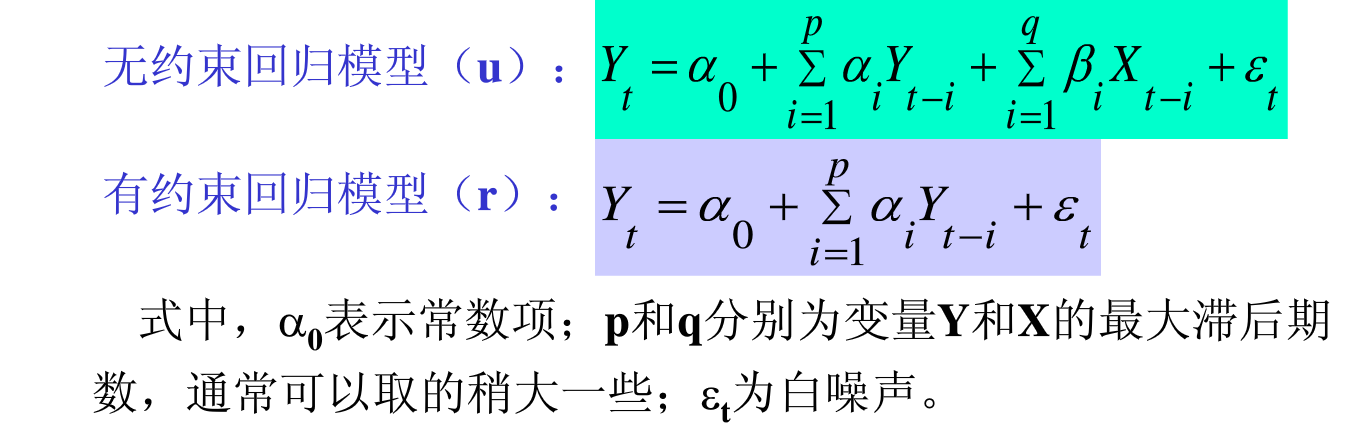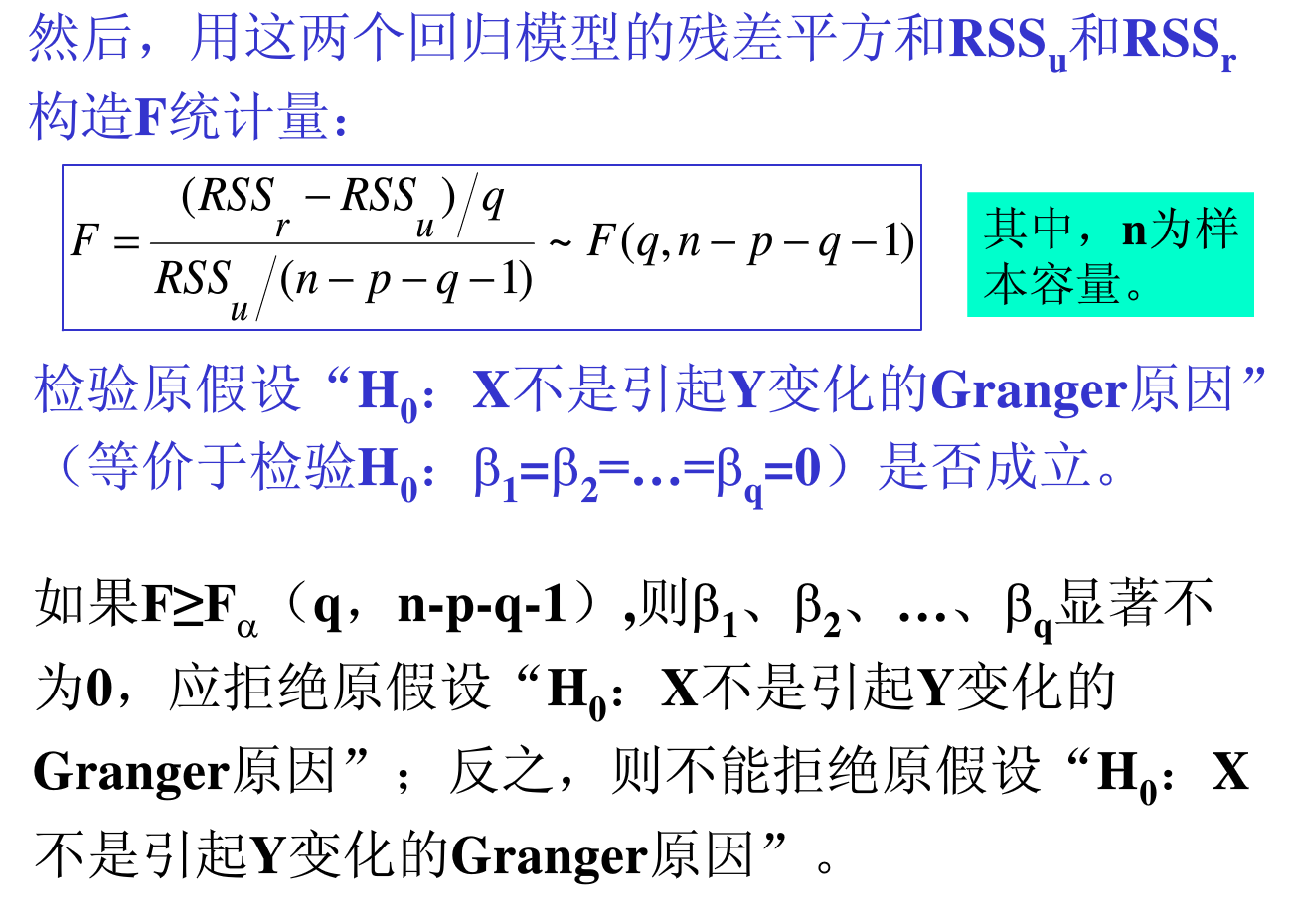VAR方法通过把系统中每一个内生变量,作为系统中所有内生变量的滞后值的函数来构造模型，从而回避了结构化模型的要求。Engle, Granger（1987）指出两个或多个非平稳时间序列的线性组合可能是平稳的。假如这样一种平稳的或的线性组合存在，这些非平稳（有单位根）时间序列之间被认为是具有协整关系的。这种平稳的线性组合被称为协整方程且可被解释为变量之间的长期均衡关系。VAR模型对于相互联系的时间序列变量系统是有效的预测模型，同时，向量自回归模型也被频繁地用于分析不同类型的随机误差项对系统变量的动态影响。如果变量之间不仅存在滞后影响，而不存在同期影响关系，则适合建立VAR模型，因为VAR模型实际上是把当期关系隐含到了随机扰动项之中。

• 定义
• 概述
• 平稳性检验
• 向量自回归模型（VAR）
• E-G检验与JJ检验
• 误差修正模型
• 脉冲响应函数
• 方差分解

## 定义

VAR模型描述在同一样本期间内的 $n$ 个变量（内生变量）可以作为它们过去值的线性函数。

$$y_{t}=c+A_{1}y_{t-1}+A_{2}y_{t-2}+\cdots +A_{p}y_{t-p}+e_{t},$$

1. $E(e_t)=0$ ,误差项的均值为 $0$
2. $E(e_{t}e_{t}’)=\Omega$, 误差项的协方差矩阵为 $\Omega$.（一个 $n*n$ 正定矩阵）
3. $E(e_{t}e_{t-k}’)=0$, （对于所有不为 $0$ 的 $k$ 都满足）,误差项不存在自相关

### 结构向量自回归

$$B_{0}y_{t}=c_{0}+B_{1}y_{t-1}+B_{2}y_{t-2}+\cdots +B_{p}y_{t-p}+\epsilon _{t},$$

## 概述

1. 滞后阶数检验，以确定最终模型的滞后阶数
2. 在滞后阶数确定后进行因果关系检验，以确定哪些序列为外生变量

• 如单位根均小于1，VAR构建完成可建立误差修正模型、脉冲响应函数及方差分解。
• 如单位根有大于1的，考虑对原始序进行降阶处理（一阶单整序列处理方法：差分或取对数，二阶单整序列：理论上可以差分与取对数同时进行，但由于序列失去了经济含义，应放弃此处理，可考虑序列的趋势分解，如分解后仍然不能满足要求，可以罢工，不建立任何模型，休息或是打砸了电脑），处理过后对新的序列（包括最初的哪些平稳序列）不断重复第一步与第二步，直至满足稳定性为止

## 平稳性检验

1. 检验平稳性，若平稳，做格兰杰检验，非平稳，作协整检验。
2. 协整检验中要用到每个序列的单整阶数。
3. 判断时间序列的数据生成过程。

VAR建模时lag intervals for endogenous要填滞后期，但是此时你并不能判断哪个滞后时最优的，因此要多次尝试，选择不同的滞后期，至 $AIC$ 或 $SC$ 最小时，所对应着的滞后为最优滞后，此时做出来的VAR模型才较为可靠。

## E-G检验与JJ检验

• EG两步法是基于回归残差的检验，可以通过建立OLS模型检验其残差平稳性

### 步骤## 误差修正模型（VEC）

VEC是有协整约束（即有长期稳定关系）的VAR模型。当变量之间存在协整关系时，可以建立ECM进一步考察短期关系